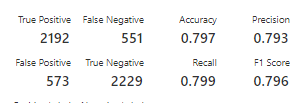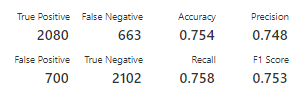# Comparing models in Azure Machine Learning

November 23, 2020

## Introduction

After discussing a few algorithms and techniques with Azure Machine Learning let us discuss techniques of comparison in Azure Machine Learning in this article. During this series of articles, we have discussed the basic cleaning techniques, feature selection techniques and Principal component analysis, etc. After discussing Regression and Classification analysis let us focus more on performing comparison in Azure Machine Learning.

## Evaluation in Azure Machine Learning

As we discussed during previous articles, we discussed limited options of evaluation using Score Model and Evaluate Model control. However, for real-world implementation score model and evaluate model controls might not be needed. Therefore, let us look at how to perform compare multiple models and different model parameters in Azure Machine Learning.

## Evaluate Model

Though we used Evaluate Model control to evaluate in the previous article, we did not use it to compare multiple models or configurations. Before looking at comparing models, let us quickly create an experiment in Azure Machine Learning to start with.

By using the AdventureWorks sample dataset the following Azure Machining Learning experiment is created as shown in the below figure.Let us quickly go through how the above experiment was created as we have done this multiple times in previous articles. As we have 28 attributes in the sample dataset, we need to reduce them to a dataset that will be valid for the prediction. From the Select Columns in Dataset, you can select the 13 attributes for the model. Then, we will perform a data split by 70/30 to train the model and the test model respectively. Then the Multiclass Decision Forest classification technique was used to train the model. In the Train Model control, the BikeBuyer column is selected as the predictable column and then by using Score Model and Evaluate Model evaluation was done. For the Regression model, the predictable column was changed to the YearlyIncome.

If you remember, when we need to compare models, we used multiple experiments therefore we had to compare them manually.

Let us say we want to compare, between Multiclass Decision Forest and Multiclass Decision Jungle classification techniques. For that, the following experiment is created.

In the above model, what we have done is, another Train Model is added and the train output of the Split Data is connected to the new Train Model. As shown in the above figure, the Multiclass Decision Jungle and the output of the Train Model are linked to the Score model along with the Test date from the Split Data control. Finally, the output of the Score Model is linked to the available Evaluate Model.

Now, let us look at the evaluate output from the Evaluate Model by right-clicking the control and selecting the Visualize option.In the evaluation results, you will have the evaluation parameters for both techniques. By comparing these two techniques on one screen, you can decide what the better technique is much easier.

Further, the output of the Evaluation Model will be different when you use different types of techniques. Let us look at what will be the output if we use Two Class classification techniques instead of Multi-Class classification techniques.

Let us look at the output of the Evaluation Model when Two-Class Decision Forest Model, Two-Class Decision Jungle algorithms are used.

As we discussed in the Classification Techniques, ROC, Precision/Recall and Lift charts are used to evaluate in two-class classification.In the ROC curve, you can see that the first model is better which is the Two-Class Decision Forest.

Now let us look at the Precision/Recall curve as shown below.In this comparison too, the better algorithm is the Two-Class Decision Forest.

Then finally, let us look at the LIFT chart.Even in the Lift chart comparison, the better algorithm is Two-Class Decision Forest by a small margin.

As we discussed in the previous article, there multiple evaluation parameters for the Two-Class classification models such as Precision, Recall and F1 Score. By clicking the blue or red key on the right side, you can compare the models.

 Two-Class Decision Forest Two-Class Decision JungleSimilar to the Two-Class classification and Multi-Class classification, we can compare the results of Regression models as well. In the regression model, we are using the YearlyIncome attribute as the predictable column. If you can remember, there are several regression techniques that were discussed in the previous article. First, let us configure the regression techniques for Evaluation in Azure Machine learning as shown below.

In the above experiment, Neural Network regression and Decision Forest Regression techniques are used to compare.

Let us analyze the evaluation parameters as we did before.

In the above comparison, you can see that the Decision Forest Regression technique is better than the Neural Network regression as it has lesser error values.

Comparision in Azure Machine Learning can be extended to different feature evaluation as well

## Evaluation of Model Parameters

As you are aware, there are model parameters that can be defined to get higher accuracy. Let us look at Neural Network Regression. The following are the default configuration for the Neural Network Regression.These parameters are the properties of Neural Network Regression. Now we need to change these parameters. However, what is the guarantee that new parameters have higher accuracy? For that, we can use the same comparison technique as we did before. Details of these parameters are given in the Further references section.

We will configure the Experminent as shown in the below figure.

As you can see, now you have the same algorithm for the comparison. But let us change the parameters of the newly added Neural Network Regression for the comparison as shown below.As you can see, we have modified the learning rate to 0.002 which is 0.001 by default. Further, we have modified the initial learning weights diameters to 0. 2and the momentum to 0.5.

Let us look at the comparison in Azure Machine Learning as we did before.As you can see from the above figure, the modified model with different parameters has improved as the error rates have gone down.

Similarly, we have done a comparison in Azure Machine Learning for the Multicast Decision Forest classification algorithm. In this scenario too, we have changed the default parameters and the following are the comparison results of those.## Feature Selection Comparison

If you can remember, during our feature selection techniques, we limit the attributes as all the attributes do not make a major contribution to higher accuracy. Let us use the comparison technique to verify those.

Let us select different columns for the comparison model. Since we have to use different data set, the same split data control cannot be used. We need to add a new Split Data Control and modify the experiment accordingly as shown below.

We have added other Select Columns in Dataset control to filter the few more attributes. Let us perform a comparison in Azure Machine Learning.Similarly, you can perform a comparison in Azure Machine Learning with the inclusion of Principal Component Analysis as we discussed before.

## Important

It is important to note that comparison can be done between similar models only. For example, you cannot compare models of two-class classification and multi-class classification algorithms as it not a valid comparison in Azure Machine Learning.

## Conclusion

In this article, we discussed how to perform comparison in Azure Machine Learning. We have used Evaluation Model to compare in the previous articles but in this article, we were able to compare two models using the same control. In this article, we saw that we can compare the accuracy of models between different models of the same class. Further, we were able to compare the different configurations of parameters of the same model. Besides, we were able to compare feature selection and Principal Component Analysis impacts as well.

## Further References

The following link provide you additional reading in neural network regression that was used to perform a comparison in Azure Machine Learning: